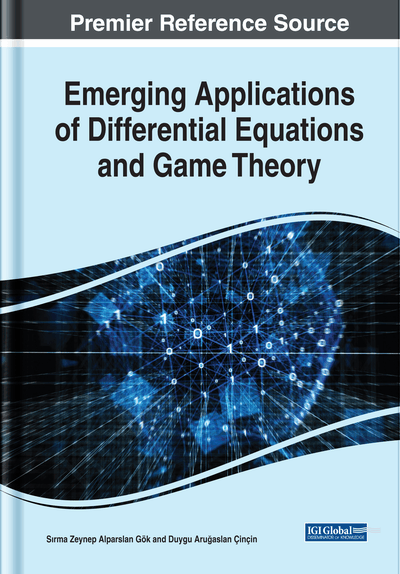The Stability of an Epidemic Model With Piecewise Constant Argument by Lyapunov-Razumikhin Method

Nur Cengiz (Süleyman Demirel University, Turkey) and Duygu Aruğaslan-Çinçin (Süleyman Demirel University, Turkey)
DOI: 10.4018/978-1-7998-0134-4.ch011
Available
\$37.50
No Current Special Offers

Abstract

The authors propose a nonlinear epidemic model by developing it with generalized piecewise constant argument (GPCA) introduced by Akhmet. The authors investigate invariance region for the considered model. For the taken model into consideration, they obtain a useful inequality concerning relation between the values of the solutions at the deviation argument and at any time for the epidemic model. The authors reach sufficient conditions for the existence and uniqueness of the solutions. Then, based on Lyapunov-Razumikhin method developed by Akhmet and Aruğaslan for the differential equations with generalized piecewise constant argument (EPCAG), sufficient conditions for the stability of the trivial equilibrium and the positive equilibrium are investigated. Thus, the theoretical results concerning the uniform stability of the equilibriums are given.
Chapter Preview
Top

Background

The mathematical evaluation of the problems encountered in real life and the interpretation of their past and future dynamics has been and continues to be a subject that attracted the attention of the scientific world. In this direction, it is possible to express these problems as mathematical models by differential equations. With the help of the qualitative theory of differential equations, informations about the behaviors of these models can be provided. However, the fact that these information presents distant results from the reality phenomenon strengthens the likelihood of misleading the behavior of real processes. In this situation, it is possible to reach the findings that will affect the life negatively by the models which do not fully reflect the dynamics of real life problems. In order to overcome this issue, it would be a step in the right direction to consider differential equations with deviation argument instead of ordinary differential equations. Because, differential equations with deviation argument improve models representing real life problems, and moreover they contribute to understanding how a situation in the past of these problems affect the current and subsequent state of them. In other words, the effects of a past value of the models that has been constructed and developed with the help of such equations on the current behavior can be observed. Therefore, the efforts to achieve the development of such equations have received considerable attention by many scientists. As a result of these efforts, the qualitative theory of such equations has shown a serious development process. It is possible to see that this process introduces differential equations which have arguments such as delay, piecewise constant argument, generalized piecewise constant argument (Cooke & Wiener, 1984; Akhmet 2007a, 2007b).

Key Terms in this Chapter

SIS: A model is a simple mathematical model of epidemics.

Population Dynamics: Population dynamics is the branch of life sciences that studies the size and age composition of populations as dynamical systems, and the biological and environmental processes driving them (such as birth and death rates, and by immigration and emigration). Example scenarios are ageing populations, population growth, or population decline.

Outbreak: Sometimes distinguished from an epidemic as more localized, or the term less likely to evoke public panic.

Epidemic: The occurrence of more cases of disease, injury or other health condition than expected in a given area or among a specific group of persons during a particular period. Usually, the cases are presumed to have a common cause or to be related to one another in some way.

GPCA: Generalized piecewise constant argument is a deviation argument which is a piecewise function considered in differential equations with piecewise constant argument of generalized type.

Complete Chapter List

Search this Book:
Reset